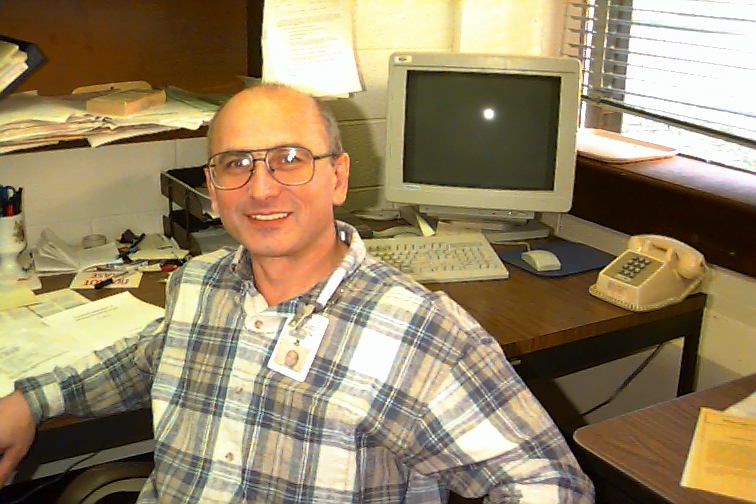ESC CODE FACT SHEET1. Code Name: Equilibrium & Stability Code (ESC)

2. Category: IIa. Fixed boundary Plasma Equilibrium

3. Responsible Physicist: Leonid E. Zakharov

5.One line description: Interactive code for massive processing experimental data and comparing with the theory.

6. Computer systems which code runs on: Any Unix machine

7. Typical running time: 1-30 sec/case

8. Approximate number of code lines: 64,000

9. Does this code read data files from another code?  From interface file, generated by EFIT

10. Does this code produce data files that can be read by another code? All the internal profiles a represented in terms of splines. So, the interface with any other code is trivial. ESC may call PEST, DCON, VMEC, JSOLVER , BALLOON. ESC is used by ASTRA (transport simulation) as an equilibrium solver. Now it is under implememtation into TRANSP as an equilibrium solver.

11. 1-2 paragraph description of code: Code uses Newton scheme  to solve the nonlinear Grad-Shafranov equation. For solving intermediate linearized Grad-Shafranov equation it uses eiter gridless (sweeping) technique  (which guarantees a  prescribed accuracy)
or the Runger-Kutta (faster) method.

12. Similar codes to this code, and distinguishing differences: Compared with JSOLVER, VMEC, ESC allows to use any combination of input profiles which determines plasma pressure and the current density.

13. Journal References describing code: L.E.Zakharov, A.Pletzer. "Theory of perturbed equilibria for solving the Grad-Shafranov
equation". Physics of Plasmas, v.6, 4693-4704 (1999).

14. New code capabilities planned for next 1-2 years: Reconstruction of NSTX equilibria from MSE and EFIT data. Low- and intermediate-n linear stability.  3-D extension of the equilibrium solver.

15. Code users: L. Zakharov

16. Present and recent applications of code: TFTR stability studies.

17. Status of code input/output documentation. Check one: ( ) does not exist ( ) incomplete ( X ) exists. 52,000 lines of documentation
maintained by a special software.

18 Year Code was first used and present frequency of use: 1994. In continued use and upgrading.

19. Estimate of Man-Years invested in developing code: 6

20. Catagories of usage of Code (Check all that apply): (X) application code to do analysis and prediction of experiments (X) numerical testbed of theoretical ideas (X) physics module to be used in integrated moddelling ( ) code for machine design

21. Language code is written in: C (95 %) and Fortran (5 %)

22. Results of intercomparisons with other codes and results of validation against experiments. Equilibrium part has been proved to be more accurate than Drozdov's POLAR equilibrium solver, JSOLVER, VMEC. In its simplest form (ideal one fluid MHD), ballooning stability part of ESC has been verified with M.Chance and A.Glasser ballooning codes. Compared with the TFTR data: 1. The code proved that widely used one-fluid theory does not fit the experiment in essential details. 2. The code revealed the role of ballooning modes in TFTR high-beta disruption triggering. 3. The code predicts appearance, frequency and range of wave numbers of so-called "Kinetic" (really MHD) ballooning modes in TFTR.